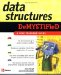A linked list is implemented differently in Java than it is in C++ because Java has a LinkedList class defined as part of the Java collection framework, which is found in the java.util package.

The Java LinkedList class performs the same basic operations that we defined in the C++ LinkedList class. In addition, the Java LinkedList class has other features used to manipulate nodes in the linked list. You ll learn about these in the next two chapters, where the LinkedList class is used to implement other kinds of data structures. For now, we ll show you how to implement a linked list using Java.

The best thing about using the Java LinkedList class is that you simply declare an instance of the LinkedList class and call member methods as required by your program to manipulate the linked list.

The code example in this section is the Java version of the C++ application described previously in this chapter. You ll notice that both programs are similar, although the Java application has some subtle differences.

The first statement in the Java program is the same statement as is in the C++ program. It declares an instance of the LinkedList class. The next three statements call the add() member method of the LinkedList class to create a new node at the end of the linked list.

The add() member method is similar to the appendNode() member function used in the C++ application. However, you probably noticed that the parameter passed to the add() method is somewhat different from the parameter passed to the appendNode() member function. The parameter to the appendNode() member function is an integer, which is a primitive data type. However, the add() member method requires an object and not a primitive data type.

As you ll remember from your Java programming class, Java has a wrapper class for primitive data types. A wrapper class definition contains a data member that is of the corresponding primitive data type and defines member methods to manipulate the data type.

This example declares an instance of the Integer wrapper class using the new operator as the parameter to the add() member method. You pass to the constructor of the Integer class the integer that you want placed in the new node. The instance of the Integer class is an object, which is what the add() member method expects to receive. All the Java collections require a data type that inherits from the object. This is somewhat similar to the void pointer in C++. You re not allowed to store primitive data types in the collections as you can in C++.

Once three nodes are appended to the linked list, the program then displays the contents of the linked list by using two for loops . The first for loop displays the linked list in natural order. This has the same effect as if you called the displayNodes() member function in the C++ program. The second for loop displays the linked list in reverse order, which is identical to calling the displayNodesReverse() member function in the C++ program.

Both for loops call the size () member method of the LinkedList class to determine the number of nodes that are contained in the linked list. The data member of each node is retrieved by calling the get() method of the LinkedList class. The get() method requires one parameter, which is the number of the node on the list. In this example, the number is the integer value of the for loop.

The get() member method returns an object that must be type cast to an instance of the Integer class.

` (Integer)list.get(i); `

The node is then assigned to an Integer object and displayed on the screen. This is similar behavior with all the Java collection classes. With JDK 1.5, type safe collections will be introduced that are similar to template classes in C++, and you ll have an option to specify the type of object in a collection so all this type casting will not be necessary. It will also allow the compiler to do the type checking, leading to fewer errors. This generic object reference has the same pitfall as the void pointer in C++: it can lead to errors.

The only difference between both for loops is the expression used to determine the direction of the loop. Otherwise, both loops are identical.

Here is the output of the following example:

10

20

30

30

20

10

` import java.util.LinkedList; public class LinkedListDemo {  public static void main(String[] args) throws IOException  {  LinkedList list = new LinkedList();  list.add(new Integer(10));  list.add(new Integer(20));  list.add(new Integer(30));  for(int i=0; i<list.size(); i++)  {  Integer temp = (Integer)list.get(i);  System.out.println(temp);  }  for(int i=list.size()-1; i>=0; i--)  {  Integer temp = (Integer)list.get(i);  System.out.println(temp);  } } } `Data Structures Demystified (Demystified)
ISBN: 0072253592
EAN: 2147483647
Year: 2006
Pages: 90

Similar book on Amazon